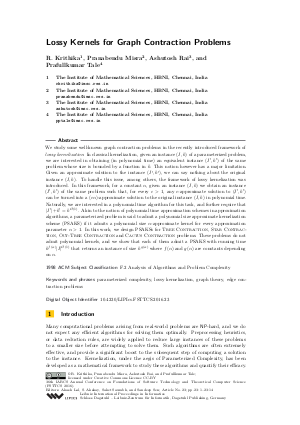Document# Lossy Kernels for Graph Contraction Problems

### Authors R. Krithika, Pranabendu Misra, Ashutosh Rai, Prafullkumar Tale## File

LIPIcs.FSTTCS.2016.23.pdf
• Filesize: 0.5 MB
• 14 pages

## Cite As

R. Krithika, Pranabendu Misra, Ashutosh Rai, and Prafullkumar Tale. Lossy Kernels for Graph Contraction Problems. In 36th IARCS Annual Conference on Foundations of Software Technology and Theoretical Computer Science (FSTTCS 2016). Leibniz International Proceedings in Informatics (LIPIcs), Volume 65, pp. 23:1-23:14, Schloss Dagstuhl - Leibniz-Zentrum für Informatik (2016)
https://doi.org/10.4230/LIPIcs.FSTTCS.2016.23

## Abstract

We study some well-known graph contraction problems in the recently introduced framework of lossy kernelization. In classical kernelization, given an instance (I,k) of a parameterized problem, we are interested in obtaining (in polynomial time) an equivalent instance (I',k') of the same problem whose size is bounded by a function in k. This notion however has a major limitation. Given an approximate solution to the instance (I',k'), we can say nothing about the original instance (I,k). To handle this issue, among others, the framework of lossy kernelization was introduced. In this framework, for a constant alpha, given an instance (I,k) we obtain an instance (I',k') of the same problem such that, for every c>1, any c-approximate solution to (I',k') can be turned into a (c*alpha)-approximate solution to the original instance (I, k) in polynomial time. Naturally, we are interested in a polynomial time algorithm for this task, and further require that |I'| + k' = k^{O(1)}. Akin to the notion of polynomial time approximation schemes in approximation algorithms, a parameterized problem is said to admit a polynomial size approximate kernelization scheme (PSAKS) if it admits a polynomial size alpha-approximate kernel for every approximation parameter alpha > 1. In this work, we design PSAKSs for Tree Contraction, Star Contraction, Out-Tree Contraction and Cactus Contraction problems. These problems do not admit polynomial kernels, and we show that each of them admit a PSAKS with running time k^{f(alpha)}|I|^{O(1)} that returns an instance of size k^{g(alpha)} where f(alpha) and g(alpha) are constants depending on alpha.
##### Keywords
• parameterized complexity
• lossy kernelization
• graph theory
• edge contraction problems

## Metrics

• Access Statistics
• Total Accesses (updated on a weekly basis)
0

## References

1. T. Asano and T. Hirata. Edge-contraction problems. Journal of Computer and System Sciences, 26(2):197-208, 1983. URL: http://dx.doi.org/10.1016/0022-0000(83)90012-0.
2. R. Belmonte, P. A. Golovach, P. van 't Hof, and D. Paulusma. Parameterized complexity of three edge contraction problems with degree constraints. Acta Informatica, 51(7):473-497, 2014. URL: http://dx.doi.org/10.1007/s00236-014-0204-z.
3. H. L. Bodlaender, S. Thomassé, and A. Yeo. Kernel bounds for disjoint cycles and disjoint paths. Theoretical Computer Science, 412(35):4570-4578, 2011. URL: http://dx.doi.org/10.1016/j.tcs.2011.04.039.
4. A. E. Brouwer and H. J. Veldman. Contractibility and NP-completeness. Journal of Graph Theory, 11(1):71-79, 1987.5. L. Cai and C. Guo. Contracting Few Edges to Remove Forbidden Induced Subgraphs, pages 97-109. Springer International Publishing, 2013. URL: http://dx.doi.org/10.1007/978-3-319-03898-8_10.
6. M. Cygan, F. V. Fomin, L. Kowalik, D. Lokshtanov, D. Marx, M. Pilipczuk, M. Pilipczuk, and S. Saurabh. Parameterized Algorithms. Springer-Verlag, 2015.7. R. Diestel. Graph Theory. Springer-Verlag Berlin Heidelberg, 2000.8. M. Dom, D. Lokshtanov, and S. Saurabh. Kernelization lower bounds through colors and IDs. ACM Transactions on Algorithms (TALG), 11(2):13, 2014.9. R. G. Downey and M. R. Fellows. Fundamentals of Parameterized Complexity. Springer-Verlag London, 2013.10. J. Flum and M. Grohe. Parameterized Complexity Theory. Springer, 2006.11. M. R. Garey and D. S. Johnson. Computers and Intractability: A Guide to the Theory of NP-Completeness. W.H.Freeman and Company, 1979.12. P. A. Golovach, M. Kamiński, D. Paulusma, and D. M. Thilikos. Increasing the minimum degree of a graph by contractions. Theoretical Computer Science, 481:74-84, 2013. URL: http://dx.doi.org/10.1016/j.tcs.2013.02.030.
13. P. A. Golovach, P. van ’t Hof, and D. Paulusma. Obtaining planarity by contracting few edges. Theoretical Computer Science, 476:38-46, 2013. URL: http://dx.doi.org/10.1016/j.tcs.2012.12.041.
14. S. Guillemot and D. Marx. A faster FPT algorithm for Bipartite Contraction. Information Processing Letters, 113(22-24):906-912, 2013. URL: http://dx.doi.org/10.1016/j.ipl.2013.09.004.
15. P. Heggernes, P. van 't Hof, D. Lokshtanov, and C. Paul. Obtaining a bipartite graph by contracting few edges. SIAM Journal on Discrete Mathematics, 27(4):2143-2156, 2013. URL: http://dx.doi.org/10.1137/130907392.
16. P. Heggernes, P. van't Hof, B. Lévêque, D. Lokshtanov, and C. Paul. Contracting Graphs to Paths and Trees. Algorithmica, 68(1):109-132, 2014.17. D. Lokshtanov, N. Misra, and S. Saurabh. On the Hardness of Eliminating Small Induced Subgraphs by Contracting Edges, pages 243-254. Springer International Publishing, 2013. URL: http://dx.doi.org/10.1007/978-3-319-03898-8_21.
18. D. Lokshtanov, F. Panolan, M. S. Ramanujan, and S. Saurabh. Lossy kernelization. CoRR, abs/1604.04111, 2016.19. B. Martin and D. Paulusma. The computational complexity of disconnected cut and 2K₂-partition. Journal of Combinatorial Theory, Series B, 111:17-37, 2015. URL: http://dx.doi.org/10.1016/j.jctb.2014.09.002.
20. T. Watanabe, T. Ae, and A. Nakamura. On the removal of forbidden graphs by edge-deletion or by edge-contraction. Discrete Applied Mathematics, 3(2):151-153, 1981. URL: http://dx.doi.org/10.1016/0166-218X(81)90039-1.
21. T. Watanabe, T. Ae, and A. Nakamura. On the NP-hardness of edge-deletion and -contraction problems. Discrete Applied Mathematics, 6(1):63-78, 1983. URL: http://dx.doi.org/10.1016/0166-218X(83)90101-4.
X

Feedback for Dagstuhl Publishing